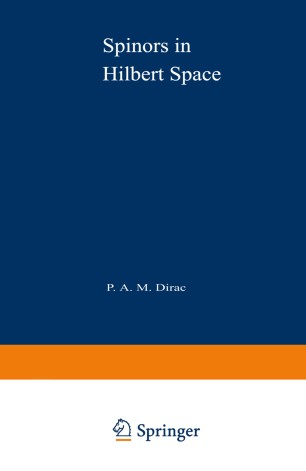# Spinors in Hilbert Space

• P. A. M. DiracBook

1. Front Matter
Pages i-1
2. P. A. M. Dirac
Pages 3-4
3. P. A. M. Dirac
Pages 5-32
4. P. A. M. Dirac
Pages 33-56
5. P. A. M. Dirac
Pages 57-91

### Introduction

1. Hilbert Space The words "Hilbert space" here will always denote what math­ ematicians call a separable Hilbert space. It is composed of vectors each with a denumerable infinity of coordinates ql' q2' Q3, .... Usually the coordinates are considered to be complex numbers and each vector has a squared length ~rIQrI2. This squared length must converge in order that the q's may specify a Hilbert vector. Let us express qr in terms of real and imaginary parts, qr = Xr + iYr' Then the squared length is l:.r(x; + y;). The x's and y's may be looked upon as the coordinates of a vector. It is again a Hilbert vector, but it is a real Hilbert vector, with only real coordinates. Thus a complex Hilbert vector uniquely determines a real Hilbert vector. The second vector has, at first sight, twice as many coordinates as the first one. But twice a denumerable in­ finity is again a denumerable infinity, so the second vector has the same number of coordinates as the first. Thus a complex Hilbert vector is not a more general kind of quantity than a real one.

### Keywords

Hilbert space complex complex number space will

#### Authors and affiliations

• P. A. M. Dirac
• 1
1. 1.Center for Theoretical StudiesUniversity of MiamiCoral GablesUSA

### Bibliographic information

• DOI https://doi.org/10.1007/978-1-4757-0034-3
• Copyright Information Springer-Verlag US 1974
• Publisher Name Springer, Boston, MA
• eBook Packages
• Print ISBN 978-1-4757-0036-7
• Online ISBN 978-1-4757-0034-3
• Buy this book on publisher's site